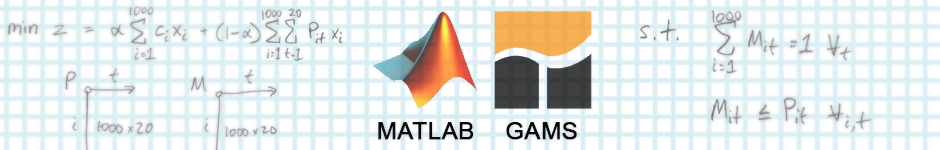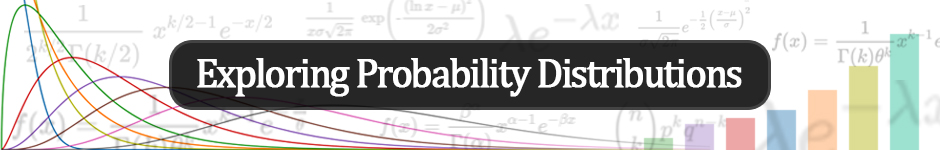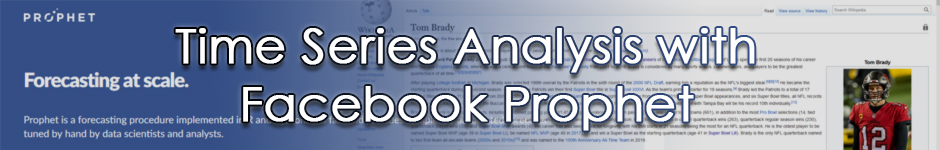## Linear Optimization with MATLAB/GAMS

Optimization with MATLAB and GAMS can aid decision-makers in the for-profit, non-profit, and public sectors.Using optimization methods requires structuring problems, identifying objectives, and imposing constraints given a problem context. Continue reading to learn how to solve optmization problems in MATLAB and GAMS.

## Exploring Probability Distributions

Probability distributions are used throughout quantitative fields where uncertainty or randomness are involved.Probabilistic thinking requires knowledge of probability distributions and the relevant statistics associated with each. Understanding these distributions can help you identify opportunities to leverage one. Continue reading to learn about the core concepts and essential distributions with examples in Python.

## Interpreting Machine Learning with SHAP

SHAP or Shapley Additive Explanations helps you understand ML models.The SHAP framework unifies the methods used to interpret and explain machine learning models. This post helps interpret and explain SHAP. Read this post to start getting into SHAP (with both high-level explanation and python example).

## Time Series Analysis with Facebook Prophet

Time series analysis is a powerful technique to generate forecasts with seasonal data.Data sets contain noise but with high-powered or elegant data science, the relevant signal can be extracted. One key technique for analysis of real-world data (primarily focused on forecasting) is time-series analysis. A popular time-series forecasting procedure is Facebook's open-source Prophet procedure. Prophet is implemented in both R and Python.